# Get a Grip on Battery Life for Your Touch-Sensitive Device

### Abstract

A highly sensitive-to-touch case changes the functionality of battery-operated medical devices, industrial meters, tablets, or cell phones with a squeeze or finger swipe. A gentle grip with these devices achieves optimum hands-on performance and battery longevity. The best solution is to optimize this sensitivity with a high-precision 24-bit ΔΣ ADC and control power consumption with effective firmware algorithms.

### Introduction

Handheld battery-operated devices such as portable medical devices, industrial meters, tablets, or cell phones provide effective tactile user interfaces through LCDs (liquid-crystal displays). Perhaps the next step in user interface for these tools is to make them sensitive to external pressures applied to their cases. With this in mind, the implementation of multiple user-programmable functions using a simple squeeze or finger swipe on a device case is promising (Figure 1).Figure 1. An infrared laser thermometer adjustable through hand pressure or touch.

For instance, by using a short squeeze, a cell phone could take a quick photograph, while a long squeeze could answer a call. This capability offers a gambit of new ways to use handheld devices, but the best of all worlds would be for the ultrasensitive exterior to have minimal impact on device battery life.

In this design solution, we will explore the two key requirements for success: precision sensitivity to touch and effective battery management techniques.

### Exterior Force Sensors Housed Inside the Device

If appropriately affixed to a handheld device, force or strain gauge sensors respond to slight changes in pressure. A proper model configuration of this gauge is a Wheatstone bridge or force sensor that consists of four resistive strain gauges.

The resistors are the modeling elements for the sensor’s strain gauges. The complete sensor construction is typically a PCB with conductive traces that have variable resistances (Figure 2).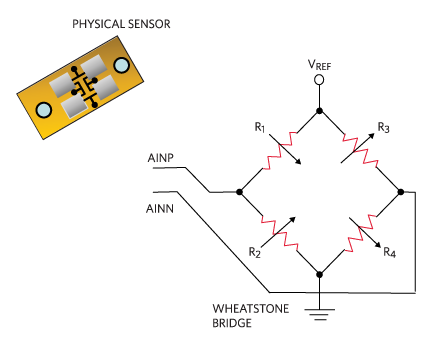Figure 2. Wheatstone bridge or force sensor.

The ideal PCB material would be a flexible plastic substrate, such as polyimide or a transparent polyester. A flexible circuit structure allows the board to conform to the desired shape or flex position without shorting the traces on the board. The generated resistor values vary with applied stress or force.

Figure 3 shows a force sensor’s arrangement of four strain gauges. In a static, stressless environment, all resister values are equal or R1 = R2 = R3 = R4. With the application of force or even a slight touch, the values of the resistors change such that R1 > R3 and R2 < R4, while R1 = R4 and R2 = R3.

Resistive magnitudes of the four bridge elements vary from 300Ω to 10kΩ. If the designer stays within the bounds of the mechanical stress restrictions of these gauges, the full-scale output, VOUT or AINP - ANIN, varies between 10mV to 100mV.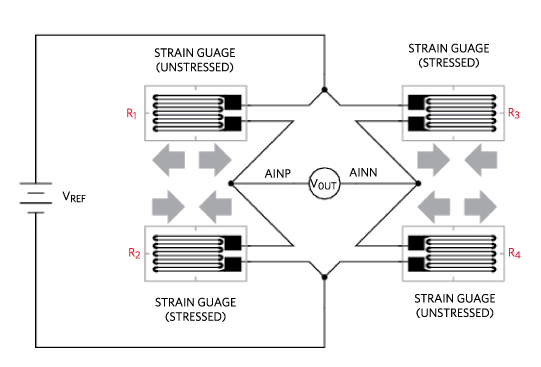Figure 3. Full-bridge flex plastic resistive strain gauge.

A resistive force sensor must be excited by a voltage source, VREF. The excitation voltage stability affects the accuracy of the measurement, making a stable voltage source necessary.

The output voltage (AINP - ANIN) of this sensor depends on the strain gauges’ physical and resistive delta range. For example, a bridge can have a resistance of 1.2kΩ, with a rated output of 2mV/V and a full-scale deflection of 0.01in. to 0.05in.

### Encircling the Entire Device

One could successfully add several bridges to the interior edges of a device. The primary challenge here is to sense the squeeze factor or the subtle changes in the device’s external package (Figure 4).

In Figure 4, the edges of the cell phone are sensitive to pressure and/or touch. Just a little squeeze excites the sensors. At this point, the device measures the sensor’s output voltages with a differential input IC.Figure 4. Multiple strain gauge bridges on the device perimeter provide squeeze-sensing capability.

### Signal Conditioning Options

A delta-sigma, analog-to-digital converter (ΔΣ ADC) is the appropriate building block for applications where capturing and digitizing small differential voltages is crucial. ?S ADCs have differential input ports to connect to these bridges. The ΔΣ ADC captures the difference between the voltages (in Figure 2, AINP and AINN) to record the applied force.

This sensor and the ΔΣ ADC require a reference voltage (VREF). Attaching VREF to the bridges and the ΔΣ ADC’s reference in Figure 5 provides convenient ratiometric digital results.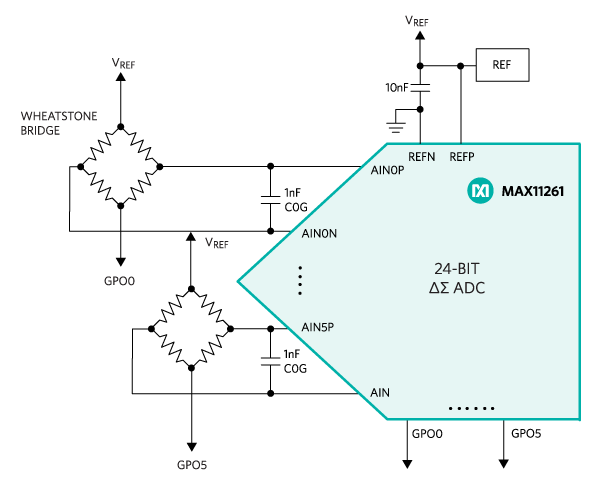Figure 5. A six-channel 24-Bit ΔΣ ADC with six programmable GPO ports.

The multi-channel ΔΣ ADC shown in Figure 5 is an appropriate fit for this application. This design requires an ADC that can convert small incremental voltage changes to a digital code. The 24-bit ΔΣ ADC generates 224 or approximately 17 million digital output codes. For an ideal 24-bit ΔΣ ADC with a 3.0V input range, the size of the least significant bit (LSB) is approximately 179nV. But in Figure 5, the ΔΣ ADC internal gain block improves this picture. For instance, a resolution of 150nVRMS with an internal gain of 64 and a 3.0V reference is achieved at a sample rate of 1ksps.

### Power Conservation

The ΔΣ ADC is a powerful addition to this circuit as it resolves very small differential bridge voltages from physical movements. Given that constant exterior monitoring is critical, a requirement in this battery-powered environment is to keep the power consumption of these sensing units as low as possible.

The power dissipation in bridge sensing circuits come from two places, the resistive bridges and the ΔΣ ADCs.

The resistive bridge power (PBRIDGE) is equal to VREF2/RBRIDGE. For example, if RBRIDGE = 1.2kΩ and VREF = 3.0V, the power dissipated by one sensor is 7.5mW (Equations 1 and 2).(Eq. 1)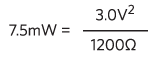(Eq. 2)

Typically, the specifications for the ΔΣ ADC current consumption are the analog (IANA) and digital (IDIG) operating supply currents. In our circuit, the analog and digital power supplies to the ΔΣ ADC are 3.3V. Using the specifications of the device in Figure 4, the ΔΣ ADC IANA = 4.2mA and IDIG = 0.7mA, which provide operating power (PADC_OP) during the channel scan time equal to 16.17mW (Equations 3 and 4).

 PADC_OP = VDD × (IANA + IDIG) (Eq. 3)

 16.17mW = 3.3V × (4.2mA+0.7mA) (Eq. 4)

The total operating power (PTOT_OP) of the ΔΣ ADC and the sensor is 23.67mW (Equations 5 and 6).

 PTOT_OPVV= = PBRIDGE + PADC_OP (Eq. 5)

 23.67mW = 7.5mW + 16.17mW (Eq. 6)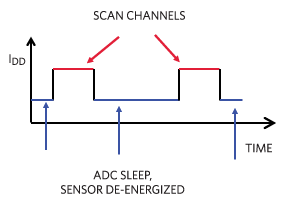Figure 6. Power conservation timing diagram.

For the ΔΣ ADC in Figure 4, if the GPOx voltages equal VREF, the bridge currents and bridge power reach a near-zero value (PBRIDGE_SL = 0W). Additionally, the typical ΔΣ ADC sleep mode current (ISL) is ～1.3µA. This produces the ΔΣ ADC sleep power (PADC_SL) equal to 4.29µW (Equations 7 and 8).

 PADC_SL= VDD × ISL (Eq. 7)

 4.29µW = 3.3V × 1.3µA (Eq. 8)

The total sleep power (PTOT_SL) of the ΔΣ ADC and the sensor are 4.29W (Equations 9 and 10).

 PTOT_SL = PBRIDGE_SL + PADC_SL (Eq. 9)

 4.29µW = 0W + 4.2µ9W (Eq. 10)

The ratio in power consumption between the operating and sleep configurations is approximately 5,517.

Now, the total power consumption of this application becomes a timing algorithm challenge. This extremely high ratio is a strong motivator to effectively control the operating and sleep modes through firmware programming. In this challenge, the designer ascertains the measurement rate requirements as the sensors measure a human event in the 1Hz to 20Hz range.

### Conclusion

The functionality of battery-operated medical devices such as industrial meters, tablets, or cell phones could be extended by making the device case highly sensitive to touch with a squeeze or finger swipe. We discussed a way to gently get a grip on these devices to achieve optimum hands-on performance and battery longevity. The best solution is to optimize measurements with a high-precision 24-bit ΔΣ ADC and control power consumption with effective firmware algorithms.